## A supertanker filled with oil has a total mass of 6.1 x108 kg. If the dimensions of the ship are those of a rectangular box 300 meters long,

Question

A supertanker filled with oil has a total mass of 6.1 x108 kg. If the dimensions of the ship are those of a rectangular box 300 meters long, 80 meters wide, and 40 meters high, determine how far the bottom of the ship is below sea level. 4. [psea 1 020 kgm3 =
a) 10 m
b) 15 m
c) 20 m
d) 25 m
e) 30 m

in progress 0
2 months 2021-07-21T12:27:59+00:00 1 Answers 2 views 0

The bottom of the sea is 25 m below sea level.

Explanation:

Given data

Mass = 6.1 ×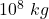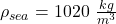We know that Buoyant force on the tank is equal to gravity force of the tank.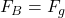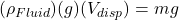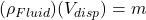1020 ×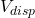= 6.1 ×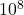= 598039.21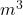We know that= W × L × H

598039.21 = 300 × 80 × H

H = 25 m

Therefore the bottom of the sea is 25 m below sea level.